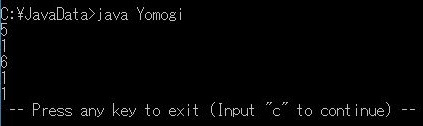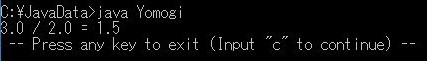# Four arithmetic operations

Add or subtract numbers, that kind of thing

・ Addition ⇒ + ・ Subtraction ⇒- ・ Multiplication ⇒ * ・ Division ⇒ / ・ Remainder of division ⇒%

Is it about "remainder of division" that is worrisome? For the time being, let's put each calculation process into the usual basic form.

#### `Yomogi.java`

``````
public class Yomogi{
public static void main(String[] args){
int a, b, result;
a = 3;
b = 2;

result = a + b;
System.out.println(result);

result = a - b;
System.out.println(result);

result = a * b;
System.out.println(result);

result = a / b;
System.out.println(result);

result = a % b;
System.out.println(result);
}
}
``````

・ Variables are integers, a, b, and result. ・ Put the calculation result in result and output ・ Once the result is output, it will be reused in the next calculation.

RunI see, the remainder (%) 3/2 = 1 ** remainder 1 ** Only this part is displayed.

Anyway It is difficult to understand even if only the calculation result is displayed. Also, division (/) is where you want to display after the decimal point.

So, next time I will make it possible to use the decimal point I want to put a character string before the calculation result as to what the calculation is.

# Use decimal point in variable

The integer was "int", but it seems to be "double" if you need the decimal point. There seems to be other "float", but I didn't notice it. Just remember when you need it.

-Floating point variable is "double"

``````double a, b, result;
a = 3.0;
b = 2.0;

result = a / b;
``````

You should be able to use the decimal point with this. I decided to execute it together with the addition of the character string ↓.

# Display ** character string ** and ** numerical value in variable ** in succession at the time of output

The goal is to produce an output like "a / b = answer" in the execution result.

-The "a / b =" part is output as a ** character string ** ・ The answer part displays the ** numerical value ** of the variable "result". -It is "** + **" to connect a character string and a variable with print or println.

• "+" Has a strong image by adding the four arithmetic operations ...

To realize these ↓ Is it like this?

``````System.out.println("3.0 / 2.0 = " + result);
``````

Put it in the usual uninflected word.

#### `Yomogi.java`

``````
public class Yomogi{
public static void main(String[] args){
double a, b, result;
a = 3.0;
b = 2.0;

result = a / b;
System.out.println("3.0 / 2.0 = " + result);
}
}
``````

-Define variables with "double" -Output by connecting ** character string ** and ** variable ** with "+" in println

RunAt the time of int, the remainder was truncated and the answer was "1" In double, it was displayed safely at "1.5".

The formula is also displayed before the calculation result, as you can imagine.

# Calculations with a mixture of integers and floating point numbers

Be careful when putting the result of integer and floating point calculation into a variable.

There are many possible situations For the time being, I decided to remember only the 6 patterns of ↓.

① Put integer and floating point results in ** floating point variables ** ⇒ ** OK **

``````double result;
result = 3 - 1.5;
``````

"1.5" is entered in "result". Does it look like it will be converted to floating point?

② Put integer and floating point results in ** integer variables ** ⇒ ** NG **

``````int result;
result = 3 - 1.5;
``````

I get an error when I run it. It doesn't seem to convert to an integer.

Here, I noticed that I had misunderstood at the time of ①. I thought I was converting from integer to floating point when I put it in a variable Was it converted to floating point when calculating? When.

So I could use variables defined with double, not variables defined with int. Isn't it?

③ Put the calculation result of integers into ** floating point variable ** ⇒ ** OK **

``````double result;
result = 3 / 2;
``````

"1.0" is entered in "result". Since it is calculated as an integer, the numbers after the decimal point are truncated.

that? After all it is converted at the timing of putting it in a variable, right? It may be converted to double both when calculating and when assigning to a variable.

④ Put the calculation result between floating point numbers in ** integer variable ** ⇒ ** NG **

``````int result;
result = 3.0 / 2.0;
``````

I get an error when I run it.

⑤ In case of (2) or (4), to put a floating-point number in ** integer variable ** If you do like ↓, it will not be an error.

``````int result;
result = (int)(3.0 - 1.5);
``````

If you add "(int)" before enclosing the formula in parentheses, it will be forcibly converted to an integer. In this case, the calculation result can be put in an integer variable (result).

However, since the decimal point is truncated when making an integer "1" is entered in "result".

⑥ It is also possible to forcibly convert the calculation result of an integer to double.

``````double result;
result = (double)(3 / 2);
``````

"1.0" is entered in "result". Since it is calculated as an integer, the numbers after the decimal point are truncated. After that, it seems to be converted to floating point.

Isn't it the same as ③? It is converted to floating point without adding "(double)". I thought, but I decided to remember it because I might use it somewhere.

This time up to here.